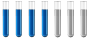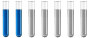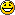## Determine the equilibrium constant

Chemistry and homework help forum.

Organic Chemistry, Analytical Chemistry, Biochemistry, Physical Chemistry, Computational Chemistry, Theoretical Chemistry, High School Chemistry, Colledge Chemistry and University Chemistry Forum.

Share your chemistry ideas, discuss chemical problems, ask for help with scientific chemistry questions, inspire others by your chemistry vision!

Please feel free to start a scientific chemistry discussion here!

Discuss chemistry homework problems with experts!

Ask for help with chemical questions and help others with your chemistry knowledge!

Moderators: expert, ChenBeier, Xen

anto007
Sr. MemberPosts: 34
Joined: Tue Aug 10, 2021 10:13 am
Location: Italy

### Determine the equilibrium constant

Hi everybody, would you help me to understand how to do this exercise:
"Determine the equilibrium constant for the following reaction at 298 K.
2 Fe3+(aq) + H2O2(aq)-->2H+(aq) + O2(g) + 2 Fe2+(aq) 📖."
Last edited by anto007 on Sun Oct 24, 2021 10:03 am, edited 1 time in total.
The mind is like a parachute, it only works if it opens. -A. Einstein
ChenBeier
Distinguished MemberPosts: 974
Joined: Wed Sep 27, 2017 7:25 am
Location: Berlin, Germany

### Re: Determine the equilibrium constant

K = cH+^2 * pO2* cFe 2+^2/(cFe 3+^2* cH2O2)
anto007
Sr. MemberPosts: 34
Joined: Tue Aug 10, 2021 10:13 am
Location: Italy

### Re: Determine the equilibrium constant

Could you please give me another hint? It's an electrochemistry exercise, I've divided the reaction into two half-reactions:

R: 2 Fe3+ + 2 e--->2Fe2+ 📖 E°= 0,77 V
O:H2O2-->O2 + 2 H+ + 2 e- 📖 E°= 0,68 V

From here I tried to apply the formula $$K=10^\frac{nE°_{(fem)}}{0,0592}$$ but I can't get the result from the book ($$1*10^3$$):
$$K=10^\frac{2(0,77-0,68)}{0,0592} =1097,84$$
where is my mistake?
The mind is like a parachute, it only works if it opens. -A. Einstein
xmaybexmemoriesx
Jr. MemberPosts: 5
Joined: Wed Oct 27, 2021 6:02 pm

### Re: Determine the equilibrium constant

I think it's just a minor oversight. You seem to have left your reaction at the "midway" point, because most equations that contain negative coefficients result in some kind of mistake or incorrect answer due to the initial reaction not being properly or fully balanced.

The first equation can be balanced:

2 Fe3+ + -1 e = 3 Fe2+
2 Fe3+ + -1 -1e = 3 Fe2+

But because there is a negative coefficient [-1 e] in this equation, it needs to be reorganized further, this time to move the corresponding compound over to the opposite side of the reaction:

2 Fe3+ = -1e + 3 Fe2+
where e = 0.77

The second equation can also be balanced:

H2O2 = O2 + 2 H+1 + 2 e-
H2O2 = O2 + 2 H
where e = 0.68

Now, plug in your value for your Nernst equation electrochemical equation, which is used to determine cell potentials at non-standard conditions. There's a relationship between E∘cell and the thermodynamics quantities such as ΔG°(Gibbs free energy) and K (the equilibrium constant). In order to accurately calculate everything, we need to know if this is under "standard conditions" (i.e. 298.15 K), because this directly affects our values we plug into our formulas and how we calculate them. If the conditions under which we are calculating is not mentioned, we are to assume that it is under "standard conditions".
We can verify the signs are correct when we realize that n and F are positive constants and that galvanic cells, which have positive cell potentials, involve spontaneous reactions. Thus, spontaneous reactions, which have ΔG < 0, must have Ecell > 0.

ΔG∘ = −nFE∘cell 📖

This provides a way to relate standard cell potentials to equilibrium constants, since:

ΔG∘ = −RT ln K
−nFE∘cell = −RT ln K
OR
E∘cell = RT/nF ln K

The negative signs for the work indicate that the electrical work is done by the system (the galvanic cell) on the surroundings versus the idea of free energy, which is defined as the energy that was available to do the work.
It's worth recalling that the charge on 1 mole of electrons (n = 1) is given by Faraday’s constant (F).

Most of the time, the electrochemical reactions are run at standard temperature (298.15 K). Collecting terms at this temperature yields:

E∘cell = RT/nF ln K = (8.314J/K⋅mol)(298.15K)/n × 96,485C/V⋅mol ln K = 0.0257V/n In K
Where n is the number of moles of electrons.

Back to the Nernst equation - for historical reasons, the logarithm in equations involving cell potentials is usually expressed using base 10 logarithms (log), which changes the constant by a factor of 2.303"

E∘cell = 0 .0592V/n[*]logK[/color][/b] 📖, where n = 3 [due to the total number of electrons (e) in the two original equations we balanced at the very top]
E∘cell = 0.0592V/n [*] logK
= 0.0592/3 [*] log10^3
= 996.621 (it equals approximately 1000 with all the proper rounding throughout every step)
Therefore: K = 10^3 [or K = 1000]

You were originally using:

K = 10 [*]nE°(fem)/0,0592[/b] 📖 - I believe that is more for stable, standardized conditions; whereas the above equations appeared to be non-standardized with no specifics and were unstable as we had to reorganize and balance them out into equilibrium prior to being able to apply any constants/formulae.

Hopefully you arrive at the answer of 1 x 10^3 = 1000, which you mentioned is the answer in your textbook.
--------------------------------------------------------------------------------------------------------
“There is scarcely any passion without struggle.” ― Albert Camus
ChenBeier
Distinguished MemberPosts: 974
Joined: Wed Sep 27, 2017 7:25 am
Location: Berlin, Germany

### Re: Determine the equilibrium constant

996.6 is also similar as 1097,84 also not accurate to 1000.
anto007
Sr. MemberPosts: 34
Joined: Tue Aug 10, 2021 10:13 am
Location: Italy

### Re: Determine the equilibrium constant

xmaybexmemoriesx wrote: Wed Oct 27, 2021 7:57 pm I think it's just a minor oversight. You seem to have left your reaction at the "midway" point, because most equations that contain negative coefficients result in some kind of mistake or incorrect answer due to the initial reaction not being properly or fully balanced.

The first equation can be balanced:

2 Fe3+ + -1 e = 3 Fe2+
2 Fe3+ + -1 -1e = 3 Fe2+

But because there is a negative coefficient [-1 e] in this equation, it needs to be reorganized further, this time to move the corresponding compound over to the opposite side of the reaction:

2 Fe3+ = -1e + 3 Fe2+
where e = 0.77

The second equation can also be balanced:

H2O2 = O2 + 2 H+1 + 2 e-
H2O2 = O2 + 2 H
where e = 0.68

Now, plug in your value for your Nernst equation electrochemical equation, which is used to determine cell potentials at non-standard conditions. There's a relationship between E∘cell and the thermodynamics quantities such as ΔG°(Gibbs free energy) and K (the equilibrium constant). In order to accurately calculate everything, we need to know if this is under "standard conditions" (i.e. 298.15 K), because this directly affects our values we plug into our formulas and how we calculate them. If the conditions under which we are calculating is not mentioned, we are to assume that it is under "standard conditions".
We can verify the signs are correct when we realize that n and F are positive constants and that galvanic cells, which have positive cell potentials, involve spontaneous reactions. Thus, spontaneous reactions, which have ΔG < 0, must have Ecell > 0.

ΔG∘ = −nFE∘cell 📖

This provides a way to relate standard cell potentials to equilibrium constants, since:

ΔG∘ = −RT ln K
−nFE∘cell = −RT ln K
OR
E∘cell = RT/nF ln K

The negative signs for the work indicate that the electrical work is done by the system (the galvanic cell) on the surroundings versus the idea of free energy, which is defined as the energy that was available to do the work.
It's worth recalling that the charge on 1 mole of electrons (n = 1) is given by Faraday’s constant (F).

Most of the time, the electrochemical reactions are run at standard temperature (298.15 K). Collecting terms at this temperature yields:

E∘cell = RT/nF ln K = (8.314J/K⋅mol)(298.15K)/n × 96,485C/V⋅mol ln K = 0.0257V/n In K
Where n is the number of moles of electrons.

Back to the Nernst equation - for historical reasons, the logarithm in equations involving cell potentials is usually expressed using base 10 logarithms (log), which changes the constant by a factor of 2.303"

E∘cell = 0 .0592V/n[*]logK[/color][/b] 📖, where n = 3 [due to the total number of electrons (e) in the two original equations we balanced at the very top]
E∘cell = 0.0592V/n [*] logK
= 0.0592/3 [*] log10^3
= 996.621 (it equals approximately 1000 with all the proper rounding throughout every step)
Therefore: K = 10^3 [or K = 1000]

You were originally using:

K = 10 [*]nE°(fem)/0,0592[/b] 📖 - I believe that is more for stable, standardized conditions; whereas the above equations appeared to be non-standardized with no specifics and were unstable as we had to reorganize and balance them out into equilibrium prior to being able to apply any constants/formulae.

Hopefully you arrive at the answer of 1 x 10^3 = 1000, which you mentioned is the answer in your textbook.
Thank you very much for your explanation, but some passages are obscure to me, perhaps I do not understand the meaning of the symbols you have used. What do you mean by this writing: [*]?
The mind is like a parachute, it only works if it opens. -A. Einstein
ChenBeier
Distinguished MemberPosts: 974
Joined: Wed Sep 27, 2017 7:25 am
Location: Berlin, Germany

### Re: Determine the equilibrium constant

* is Multiplikation, nothing special
anto007
Sr. MemberPosts: 34
Joined: Tue Aug 10, 2021 10:13 am
Location: Italy

### Re: Determine the equilibrium constantthanks
The mind is like a parachute, it only works if it opens. -A. Einstein
xmaybexmemoriesx
Jr. MemberPosts: 5
Joined: Wed Oct 27, 2021 6:02 pm

### Re: Determine the equilibrium constant

ChenBeier wrote: Wed Oct 27, 2021 10:21 pm 996.6 is also similar as 1097,84 also not accurate to 1000.
The general Nernst Equation:

E = Eo-(RT/nF)lnQ 📖

where:
Eo = standard electrochemical cell potential (voltage)
R = ideal gas constant
T = temperature
n = moles of electrons
F = Faraday constant = 96,485 C mol-1
Q = mass-action expression (approximated by the equilibrium expression)

The standard Nernst Equation can then be simplified to:

E = Eo-(0.0592/n)log10Q 📖

where:
Eo = standard electrochemical cell potential (voltage)
n = moles of electrons
Q = mass-action expression (approximated by the equilibrium expression)

For a standard system at equilibrium E = 0 and the Nernst Equation can be simplified further to:

Eo = (0.0592/n)log10K 📖

where:
Eo = standard electrochemical cell potential (voltage)
n = moles of electrons
K = equilibrium expression

Now, we want to calculate the equilibrium constant for the two fully balanced reactions (using a number of different formulas - I show two, but ultimately end using only one) from the very beginning:

2 Fe3+ = -1e + 3 Fe2+ 📖
where e = 0.77

AND

H2O2 = O2 + 2 H 📖
where e = 0.68

The Nernst Equation:
E = Eo-(0.0592/n)log10Q 📖

Here's the Nernst Equation written for the equilibrium expression:
At equilibrium Q = K and E = 0
Eo = (0.0592/n)log10K 📖

Calculate Eo for the equations above (they are both given to us):
1st equation, Eo = 0.77V
2nd equation, Eo = 0.68V
Together, the total Eo = 1.45
As Eo > 0, the reaction should occur:

2Fe(OH)2 + 2H2O + 2OH- → 2Fe(OH)3 + 2H2O + 2e- (oxidization)
H2O2 + 2H2O + 2e- → 2H2O + 2OH- (reduction)
2Fe(OH)2 + H2O2 + 4H2O + 2OH- + 2e- → 2Fe(OH)3 + 4H2O + 2OH- + 2e- (adding all reactants together)
2Fe(OH)2 + H2O2 → 2Fe(OH)3 (simplified and balanced full equation)
OR the "overall equation": 2Fe2+ (aq) + H2O2 (aq) + 2H+ (aq) → 2H2O (l)+ 2Fe3+(aq)
Note: Since H2O2 is a stronger oxidant than Fe3+. Therefore, H2O2 will be reduced.

Calculate n (number of moles of electrons transferred):
n = 2

Now substitute values for Eo and n using this equation: logK = nE˚/0.0592 📖
where n = 2(from the balanced equations we can see that 2 electrons are transferred) and Eo = 1.45V (from the info we were given 0.77V + 0.68V = 1.45V):

logK = 2(1.45V)/0.0592
= 2.9 OR 3 (rounded)
K = 10^3 OR 1000
--------------------------------------------------------------------------------------------------------
“There is scarcely any passion without struggle.” ― Albert Camus
xmaybexmemoriesx
Jr. MemberPosts: 5
Joined: Wed Oct 27, 2021 6:02 pm

### Re: Determine the equilibrium constant

anto007 wrote: Thu Oct 28, 2021 3:06 am
xmaybexmemoriesx wrote: Wed Oct 27, 2021 7:57 pm I think it's just a minor oversight. You seem to have left your reaction at the "midway" point, because most equations that contain negative coefficients result in some kind of mistake or incorrect answer due to the initial reaction not being properly or fully balanced.

The first equation can be balanced:

2 Fe3+ + -1 e = 3 Fe2+
2 Fe3+ + -1 -1e = 3 Fe2+

But because there is a negative coefficient [-1 e] in this equation, it needs to be reorganized further, this time to move the corresponding compound over to the opposite side of the reaction:

2 Fe3+ = -1e + 3 Fe2+
where e = 0.77

The second equation can also be balanced:

H2O2 = O2 + 2 H+1 + 2 e-
H2O2 = O2 + 2 H
where e = 0.68

Now, plug in your value for your Nernst equation electrochemical equation, which is used to determine cell potentials at non-standard conditions. There's a relationship between E∘cell and the thermodynamics quantities such as ΔG°(Gibbs free energy) and K (the equilibrium constant). In order to accurately calculate everything, we need to know if this is under "standard conditions" (i.e. 298.15 K), because this directly affects our values we plug into our formulas and how we calculate them. If the conditions under which we are calculating is not mentioned, we are to assume that it is under "standard conditions".
We can verify the signs are correct when we realize that n and F are positive constants and that galvanic cells, which have positive cell potentials, involve spontaneous reactions. Thus, spontaneous reactions, which have ΔG < 0, must have Ecell > 0.

ΔG∘ = −nFE∘cell 📖

This provides a way to relate standard cell potentials to equilibrium constants, since:

ΔG∘ = −RT ln K
−nFE∘cell = −RT ln K
OR
E∘cell = RT/nF ln K

The negative signs for the work indicate that the electrical work is done by the system (the galvanic cell) on the surroundings versus the idea of free energy, which is defined as the energy that was available to do the work.
It's worth recalling that the charge on 1 mole of electrons (n = 1) is given by Faraday’s constant (F).

Most of the time, the electrochemical reactions are run at standard temperature (298.15 K). Collecting terms at this temperature yields:

E∘cell = RT/nF ln K = (8.314J/K⋅mol)(298.15K)/n × 96,485C/V⋅mol ln K = 0.0257V/n In K
Where n is the number of moles of electrons.

Back to the Nernst equation - for historical reasons, the logarithm in equations involving cell potentials is usually expressed using base 10 logarithms (log), which changes the constant by a factor of 2.303"

E∘cell = 0 .0592V/n[*]logK[/color][/b] 📖, where n = 3 [due to the total number of electrons (e) in the two original equations we balanced at the very top]
E∘cell = 0.0592V/n [*] logK
= 0.0592/3 [*] log10^3
= 996.621 (it equals approximately 1000 with all the proper rounding throughout every step)
Therefore: K = 10^3 [or K = 1000]

You were originally using:

K = 10 [*]nE°(fem)/0,0592[/b] 📖 - I believe that is more for stable, standardized conditions; whereas the above equations appeared to be non-standardized with no specifics and were unstable as we had to reorganize and balance them out into equilibrium prior to being able to apply any constants/formulae.

Hopefully you arrive at the answer of 1 x 10^3 = 1000, which you mentioned is the answer in your textbook.
Thank you very much for your explanation, but some passages are obscure to me, perhaps I do not understand the meaning of the symbols you have used. What do you mean by this writing: [*]?
I've just added a detailed explanation, with formulae and calculations, for the equilibrium constant (K). I've obtained the textbook answer of 10^3 = 1000Hopefully this helps. It was just a matter of manipulating the formula that you initially posted, so that it would be easier to utilize with the information given to you in your original electrochem problem. This is the formula I used at the end to calculate for the equilibrium constant, K:

log K = nE˚/0.0592
--------------------------------------------------------------------------------------------------------
“There is scarcely any passion without struggle.” ― Albert Camus
ChenBeier
Distinguished MemberPosts: 974
Joined: Wed Sep 27, 2017 7:25 am
Location: Berlin, Germany

Excellent post